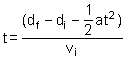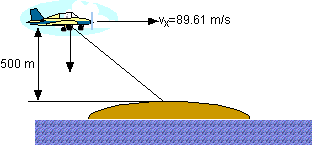+ Text Only Site
+ Non-Flash Version
+ Contact GlennAircraft Motion (Level 2) Answers You are the pilot of an airplane sent to drop supplies to victims of an accident that are stranded on a small island. The plane's altitude is always 500 meters and your speed is 89.61 m/s. Once released, how much time will elapse before the supply package reaches the level of the island? (Hint: Use the distance equation in the y-direction.)  Step 1: di = 500 m; a = -9.8 m/s2 (gravity, negative sign indicates downward direction); df = 0 m; vi = 0 m/s where di = initial distance and df = final distance Step 2: Solve the equation df = ( 1 / 2 ) at2 + vit + di for t. So,Will the descent time of the supply package change if the airplane's speed changes? (a) Yes (b) No   At what (horizontal) distance in front of the island should the package be released in order to hit the island? Step 1: During descent the package moves forward a horizontal distance of dx. v = 89.61 m/s; t = 10.10 sec Step 2: dx = vt = 905.06 m. The airplane must release the package at least 905.06 m in front of the island. What is the package's horizontal speed when it reaches the level of the island?  Neglecting air resistance, it remains constant. vx = 89.61 m/s   What is the package's vertical speed when it reaches the level of the island? Step 1: vi = 0 m/s; a = -9.8 m/s2; t = 10.10 sec Step 2: Solve the equation a = (vf - vi )/t for vf. So, vf = at + vi = -98.98 m/s ( vf = vy ) What will be the package's flight angle with respect to the level of the island as it descends? Step 1: Draw the diagram,Step 2: vy = -98.98 m/s; vx = 89.61 m/s Step 3: tan Q = vy / vx; Q = tan-1(vy / vx) = 47.84 o Suppose the victims on the island can retrieve supply packages that land within 30 meters of the island. The length of the island is 50 meters along the direction you are approaching.   How far in front of the island would the airplane have to release the supply package for it to land 30 meters in front of the island? dx(front) = distance from problem #3 + 30 m = 935.06 m   How far in front of the island would the airplane have to release the supply package for it to land 30 meters in back of the island? dx(back) = distance from problem #3 - 50 m - 30 m = 825.06 m   Time1 will be the time on your watch when you release the package and it lands 30 meters in front of the island. Time2 will be the time on your watch when you release the package and it lands 30 meters in back of the island. Calculate the amount of time you have to successfully drop the package, namely, time1- time2. Step 1: time1 = 0 sec; vx = 89.61 m/s Step 2: Solve the equation dx = (vx )(time2) for time2. Also realize that dx = dx(front) - dx(back). time2 = [ dx(front) - dx(back) ] / vx = 1.23 sec. Therefore, time1 - time2 = 1.23 sec What could be done to decrease the package's speed when it reaches the ground? Use a parachute.

Related Pages:
Standards
Activity
Worksheet
Lesson Index
Aerodynamics Index+ Inspector General Hotline + Equal Employment Opportunity Data Posted Pursuant to the No Fear Act + Budgets, Strategic Plans and Accountability Reports + Freedom of Information Act + The President's Management Agenda + NASA Privacy Statement, Disclaimer, and Accessibility CertificationEditor: Tom Benson NASA Official: Tom Benson Last Updated: Thu, May 13 02:38:26 PM EDT 2021 + Contact Glenn